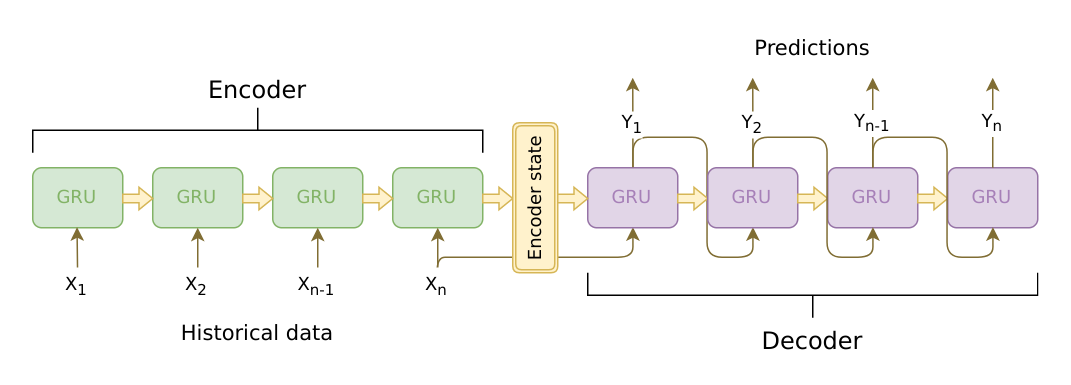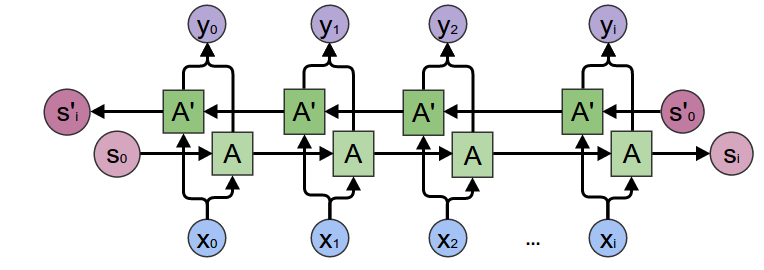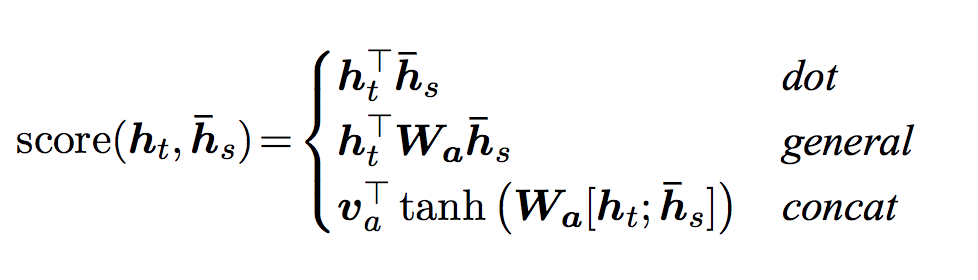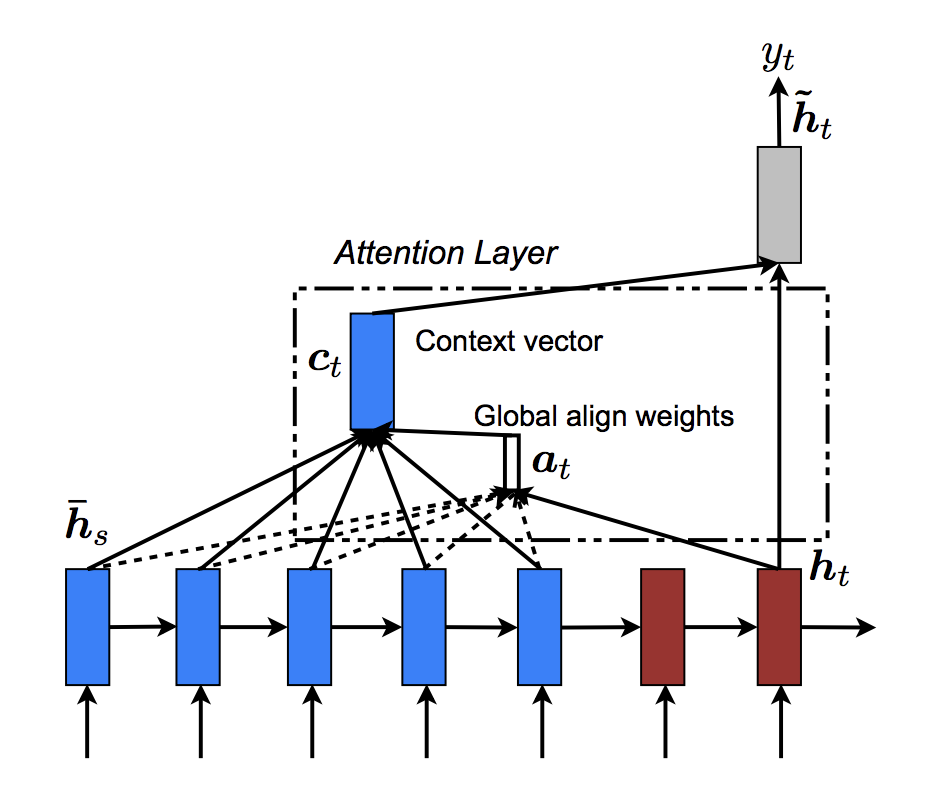# 使用PyTorch实现Chatbot

Posted by lili on February 14, 2019

## 效果

> hello?
Bot: hello .
> where am I?
Bot: you re in a hospital .
> who are you?
Bot: i m a lawyer .
> how are you doing?
Bot: i m fine .
> are you my friend?
Bot: no .
> you're under arrest
> i'm just kidding
Bot: i m sorry .
> where are you from?
Bot: san francisco .
> it's time for me to leave
Bot: i know .
> goodbye
Bot: goodbye .


## 准备

from __future__ import absolute_import
from __future__ import division
from __future__ import print_function
from __future__ import unicode_literals

import torch
from torch.jit import script, trace
import torch.nn as nn
from torch import optim
import torch.nn.functional as F
import csv
import random
import re
import os
import unicodedata
import codecs
from io import open
import itertools
import math

USE_CUDA = torch.cuda.is_available()
device = torch.device("cuda" if USE_CUDA else "cpu")


## 加载和预处理数据

Cornell电影对话语料库是电影人物的对话数据，它包括：

• 10,292对电影人物(一部电影有多个人物，他们两两之间可能存在对话)的220,579个对话
• 617部电影的9,035个人物
• 总共304,713个utterance(utterance是对话中的语音片段，不一定是完整的句子)

corpus_name = "cornell movie-dialogs corpus"
corpus = os.path.join("data", corpus_name)

def printLines(file, n=10):
with open(file, 'rb') as datafile:
for line in lines[:n]:
print(line)

printLines(os.path.join(corpus, "movie_lines.txt"))


b'L1045 +++$+++ u0 +++$+++ m0 +++$+++ BIANCA +++$+++ They do not!\n'
b'L1044 +++$+++ u2 +++$+++ m0 +++$+++ CAMERON +++$+++ They do to!\n'
b'L985 +++$+++ u0 +++$+++ m0 +++$+++ BIANCA +++$+++ I hope so.\n'
b'L984 +++$+++ u2 +++$+++ m0 +++$+++ CAMERON +++$+++ She okay?\n'
b"L925 +++$+++ u0 +++$+++ m0 +++$+++ BIANCA +++$+++ Let's go.\n"
b'L924 +++$+++ u2 +++$+++ m0 +++$+++ CAMERON +++$+++ Wow\n'
b"L872 +++$+++ u0 +++$+++ m0 +++$+++ BIANCA +++$+++ Okay -- you're gonna need to learn how to lie.\n"
b'L871 +++$+++ u2 +++$+++ m0 +++$+++ CAMERON +++$+++ No\n'
b'L870 +++$+++ u0 +++$+++ m0 +++$+++ BIANCA +++$+++ I\'m kidding.  You know how sometimes you just become this "persona"?  And you don\'t know how to quit?\n'
b'L869 +++$+++ u0 +++$+++ m0 +++$+++ BIANCA +++$+++ Like my fear of wearing pastels?\n'



data/cornell movie-dialogs corpus$head movie_conversations.txt u0 +++$+++ u2 +++$+++ m0 +++$+++ ['L194', 'L195', 'L196', 'L197']
u0 +++$+++ u2 +++$+++ m0 +++$+++ ['L198', 'L199'] u0 +++$+++ u2 +++$+++ m0 +++$+++ ['L200', 'L201', 'L202', 'L203']
u0 +++$+++ u2 +++$+++ m0 +++$+++ ['L204', 'L205', 'L206'] u0 +++$+++ u2 +++$+++ m0 +++$+++ ['L207', 'L208']
u0 +++$+++ u2 +++$+++ m0 +++$+++ ['L271', 'L272', 'L273', 'L274', 'L275'] u0 +++$+++ u2 +++$+++ m0 +++$+++ ['L276', 'L277']
u0 +++$+++ u2 +++$+++ m0 +++$+++ ['L280', 'L281'] u0 +++$+++ u2 +++$+++ m0 +++$+++ ['L363', 'L364']
u0 +++$+++ u2 +++$+++ m0 +++$+++ ['L365', 'L366']  每一行用”+++$+++”分割成4列，第一列表示第一个人物的ID，第二列表示第二个人物的ID，第三列表示电影的ID，第四列表示这两个人物在这部电影中的一段对话，比如第一行的表示人物u0和u2在电影m0中的一段对话包含ID为L194、L195、L196和L197的4个utterance。注意：两个人物在一部电影中会有多段对话，中间可能穿插其他人之间的对话，而且即使中间没有其他人说话，这两个人物对话的内容从语义上也可能是属于不同的对话(话题)。所以我们看到第二行还是u0和u2在电影m0中的对话，它包含L198和L199两个utterance，L198是紧接着L197之后的，但是它们属于两个对话(话题)。

### 数据处理

• loadLinesmovie_lines.txt 文件切分成 (lineID, characterID, movieID, character, text)
• loadConversations 把上面的行group成一个个多轮的对话
• extractSentencePairs 从上面的每个对话中抽取句对
# 把每一行都parse成一个dict，key是lineID、characterID、movieID、character和text
# 分别代表这一行的ID、人物ID、电影ID，人物名称和文本。
# 最终输出一个dict，key是lineID，value是一个dict。
# value这个dict的key是lineID、characterID、movieID、character和text
lines = {}
with open(fileName, 'r', encoding='iso-8859-1') as f:
for line in f:
values = line.split(" +++$+++ ") # 抽取fields lineObj = {} for i, field in enumerate(fields): lineObj[field] = values[i] lines[lineObj['lineID']] = lineObj return lines # 根据movie_conversations.txt文件和上输出的lines，把utterance组成对话。 # 最终输出一个list，这个list的每一个元素都是一个dict， # key分别是character1ID、character2ID、movieID和utteranceIDs。 # 分别表示这对话的第一个人物的ID，第二个的ID，电影的ID以及它包含的utteranceIDs # 最后根据lines，还给每一行的dict增加一个key为lines，其value是个list， # 包含所有utterance(上面得到的lines的value) def loadConversations(fileName, lines, fields): conversations = [] with open(fileName, 'r', encoding='iso-8859-1') as f: for line in f: values = line.split(" +++$+++ ")
# 抽取fields
convObj = {}
for i, field in enumerate(fields):
convObj[field] = values[i]
# convObj["utteranceIDs"]是一个字符串，形如['L198', 'L199']
# 我们用eval把这个字符串变成一个字符串的list。
lineIds = eval(convObj["utteranceIDs"])
# 根据lineIds构造一个数组，根据lineId去lines里检索出存储utterance对象。
convObj["lines"] = []
for lineId in lineIds:
convObj["lines"].append(lines[lineId])
conversations.append(convObj)
return conversations

# 从对话中抽取句对
# 假设一段对话包含s1,s2,s3,s4这4个utterance
# 那么会返回3个句对：s1-s2,s2-s3和s3-s4。
def extractSentencePairs(conversations):
qa_pairs = []
for conversation in conversations:
# 遍历对话中的每一个句子，忽略最后一个句子，因为没有答案。
for i in range(len(conversation["lines"]) - 1):
inputLine = conversation["lines"][i]["text"].strip()
targetLine = conversation["lines"][i+1]["text"].strip()
# 如果有空的句子就去掉
if inputLine and targetLine:
qa_pairs.append([inputLine, targetLine])
return qa_pairs


# 定义新的文件
datafile = os.path.join(corpus, "formatted_movie_lines.txt")

delimiter = '\t'
# 对分隔符delimiter进行decode，这里对tab进行decode结果并没有变
delimiter = str(codecs.decode(delimiter, "unicode_escape"))

# 初始化dict lines，list conversations以及前面我们介绍过的field的id数组。
lines = {}
conversations = []
MOVIE_LINES_FIELDS = ["lineID", "characterID", "movieID", "character", "text"]
MOVIE_CONVERSATIONS_FIELDS = ["character1ID", "character2ID", "movieID", "utteranceIDs"]

print("\nProcessing corpus...")
lines, MOVIE_CONVERSATIONS_FIELDS)

# 输出到一个新的csv文件
print("\nWriting newly formatted file...")
with open(datafile, 'w', encoding='utf-8') as outputfile:
writer = csv.writer(outputfile, delimiter=delimiter, lineterminator='\n')
# 使用extractSentencePairs从conversations里抽取句对。
for pair in extractSentencePairs(conversations):
writer.writerow(pair)

# 输出一些行用于检查
print("\nSample lines from file:")
printLines(datafile)


b"Can we make this quick?  Roxanne Korrine and Andrew Barrett are having an incredibly horrendous public break- up on the quad.  Again.\tWell, I thought we'd start with pronunciation, if that's okay with you.\n"
b"Well, I thought we'd start with pronunciation, if that's okay with you.\tNot the hacking and gagging and spitting part.  Please.\n"
b"Not the hacking and gagging and spitting part.  Please.\tOkay... then how 'bout we try out some French cuisine.  Saturday?  Night?\n"
b"You're asking me out.  That's so cute. What's your name again?\tForget it.\n"
b"No, no, it's my fault -- we didn't have a proper introduction ---\tCameron.\n"
b"Cameron.\tThe thing is, Cameron -- I'm at the mercy of a particularly hideous breed of loser.  My sister.  I can't date until she does.\n"
b"The thing is, Cameron -- I'm at the mercy of a particularly hideous breed of loser.  My sister.  I can't date until she does.\tSeems like she could get a date easy enough...\n"
b'Why?\tUnsolved mystery.  She used to be really popular when she started high school, then it was just like she got sick of it or something.\n'
b"Unsolved mystery.  She used to be really popular when she started high school, then it was just like she got sick of it or something.\tThat's a shame.\n"
b'Gosh, if only we could find Kat a boyfriend...\tLet me see what I can do.\n'


### 创建词典

# 预定义的token
SOS_token = 1  # 句子的开始
EOS_token = 2  # 句子的结束

class Voc:
def __init__(self, name):
self.name = name
self.trimmed = False
self.word2index = {}
self.word2count = {}
self.num_words = 3  # 目前有SOS, EOS, PAD这3个token。

for word in sentence.split(' '):

if word not in self.word2index:
self.word2index[word] = self.num_words
self.word2count[word] = 1
self.index2word[self.num_words] = word
self.num_words += 1
else:
self.word2count[word] += 1

# 删除频次小于min_count的token
def trim(self, min_count):
if self.trimmed:
return
self.trimmed = True

keep_words = []

for k, v in self.word2count.items():
if v >= min_count:
keep_words.append(k)

print('keep_words {} / {} = {:.4f}'.format(
len(keep_words), len(self.word2index), len(keep_words) / len(self.word2index)
))

# 重新构造词典
self.word2index = {}
self.word2count = {}
self.num_words = 3 # Count default tokens

# 重新构造后词频就没有意义了(都是1)
for word in keep_words:


MAX_LENGTH = 10  # 句子最大长度是10个词(包括EOS等特殊词)

# 把Unicode字符串变成ASCII
# 参考https://stackoverflow.com/a/518232/2809427
def unicodeToAscii(s):
return ''.join(
c for c in unicodedata.normalize('NFD', s)
if unicodedata.category(c) != 'Mn'
)

def normalizeString(s):
# 变成小写、去掉前后空格，然后unicode变成ascii
s = unicodeToAscii(s.lower().strip())
# 在标点前增加空格，这样把标点当成一个词
s = re.sub(r"([.!?])", r" \1", s)
# 字母和标点之外的字符都变成空格
s = re.sub(r"[^a-zA-Z.!?]+", r" ", s)
# 因为把不用的字符都变成空格，所以可能存在多个连续空格
# 下面的正则替换把多个空格变成一个空格，最后去掉前后空格
s = re.sub(r"\s+", r" ", s).strip()
return s

# 读取问答句对并且返回Voc词典对象
# 文件每行读取到list lines中。
lines = open(datafile, encoding='utf-8').\
# 每行用tab切分成问答两个句子，然后调用normalizeString函数进行处理。
pairs = [[normalizeString(s) for s in l.split('\t')] for l in lines]
voc = Voc(corpus_name)
return voc, pairs

def filterPair(p):
return len(p.split(' ')) < MAX_LENGTH and len(p.split(' ')) < MAX_LENGTH

# 过滤太长的句对
def filterPairs(pairs):
return [pair for pair in pairs if filterPair(pair)]

# 使用上面的函数进行处理，返回Voc对象和句对的list
print("Start preparing training data ...")
pairs = filterPairs(pairs)
print("Trimmed to {!s} sentence pairs".format(len(pairs)))
print("Counting words...")
for pair in pairs:
print("Counted words:", voc.num_words)
return voc, pairs

# save_dir = os.path.join("data", "save")
voc, pairs = loadPrepareData(corpus, corpus_name, datafile)
# 输出一些句对
print("\npairs:")
for pair in pairs[:10]:
print(pair)


Start preparing training data ...
Trimmed to 64271 sentence pairs
Counting words...
Counted words: 18008


1) 使用voc.trim函数去掉频次低于MIN_COUNT 的词。

2) 去掉包含低频词的句子(只保留这样的句子——每一个词都是高频的，也就是在voc中出现的)

MIN_COUNT = 3    # 阈值为3

def trimRareWords(voc, pairs, MIN_COUNT):
# 去掉voc中频次小于3的词
voc.trim(MIN_COUNT)
# 保留的句对
keep_pairs = []
for pair in pairs:
input_sentence = pair
output_sentence = pair
keep_input = True
keep_output = True
# 检查问题
for word in input_sentence.split(' '):
if word not in voc.word2index:
keep_input = False
break
# 检查答案
for word in output_sentence.split(' '):
if word not in voc.word2index:
keep_output = False
break

# 如果问题和答案都只包含高频词，我们才保留这个句对
if keep_input and keep_output:
keep_pairs.append(pair)

print("Trimmed from {} pairs to {}, {:.4f} of total".format(len(pairs),
len(keep_pairs), len(keep_pairs) / len(pairs)))
return keep_pairs

# 实际进行处理
pairs = trimRareWords(voc, pairs, MIN_COUNT)


keep_words 7823 / 18005 = 0.4345
Trimmed from 64271 pairs to 53165, 0.8272 of total


18005个词之中，频次大于等于3的只有43%，去掉低频的57%的词之后，保留的句子为53165，占比为82%。

### 为模型准备数据inputVar函数把batch个句子padding后变成一个LongTensor，大小是(max_length, batch)，同时会返回一个大小是batch的list lengths，说明每个句子的实际长度，这个参数后面会传给PyTorch，从而在forward和backward计算的时候使用实际的长度。

outputVar函数和inputVar类似，但是它输出的第二个参数不是lengths，而是一个大小为(max_length, batch)的mask矩阵(tensor)，某位是0表示这个位置是padding，1表示不是padding，这样做的目的是后面计算方便。当然这两种表示是等价的，只不过lengths表示更加紧凑，但是计算起来不同方便，而mask矩阵和outputVar直接相乘就可以把padding的位置给mask(变成0)掉，这在计算loss时会非常方便。

batch2TrainData 则利用上面的两个函数把一个batch的句对处理成合适的输入和输出Tensor。

# 把句子的词变成ID
def indexesFromSentence(voc, sentence):
return [voc.word2index[word] for word in sentence.split(' ')] + [EOS_token]

return list(itertools.zip_longest(*l, fillvalue=fillvalue))

m = []
for i, seq in enumerate(l):
m.append([])
for token in seq:
m[i].append(0)
else:
m[i].append(1)
return m

# lengths是一个list，长度为(batch,)，表示每个句子的实际长度。
def inputVar(l, voc):
indexes_batch = [indexesFromSentence(voc, sentence) for sentence in l]
lengths = torch.tensor([len(indexes) for indexes in indexes_batch])

def outputVar(l, voc):
indexes_batch = [indexesFromSentence(voc, sentence) for sentence in l]
max_target_len = max([len(indexes) for indexes in indexes_batch])

# 处理一个batch的pair句对
def batch2TrainData(voc, pair_batch):
# 按照句子的长度(词数)排序
pair_batch.sort(key=lambda x: len(x.split(" ")), reverse=True)
input_batch, output_batch = [], []
for pair in pair_batch:
input_batch.append(pair)
output_batch.append(pair)
inp, lengths = inputVar(input_batch, voc)
output, mask, max_target_len = outputVar(output_batch, voc)
return inp, lengths, output, mask, max_target_len

# 示例
small_batch_size = 5
batches = batch2TrainData(voc, [random.choice(pairs) for _ in range(small_batch_size)])
input_variable, lengths, target_variable, mask, max_target_len = batches

print("input_variable:", input_variable)
print("lengths:", lengths)
print("target_variable:", target_variable)
print("max_target_len:", max_target_len)


input_variable: tensor(
[[   92,   101,    76,    50,    34],
[    7,   250,    37,     6,     4],
[  123,   279,   628,     2,     2],
[   40,    75,     4,     0,     0],
[  359,    53,  7216,     0,     0],
[ 2763,   217,     4,     0,     0],
[  637,     4,     2,     0,     0],
[    6,     2,     0,     0,     0],
[    2,     0,     0,     0,     0]])
lengths: tensor([ 9,  8,  7,  3,  3])
target_variable: tensor(
[[   25,    34,   404,     7,    25],
[  283,     4,    76,    24,  1464],
[   25,     2,    37,     4,    70],
[   72,     0,  7217,     2,  1465],
[  829,     0,     4,     0,     6],
[  234,     0,     2,     0,     2],
[    4,     0,     0,     0,     0],
[    2,     0,     0,     0,     0]])
[[ 1,  1,  1,  1,  1],
[ 1,  1,  1,  1,  1],
[ 1,  1,  1,  1,  1],
[ 1,  0,  1,  1,  1],
[ 1,  0,  1,  0,  1],
[ 1,  0,  1,  0,  1],
[ 1,  0,  0,  0,  0],
[ 1,  0,  0,  0,  0]], dtype=torch.uint8)
max_target_len: 8


## 定义模型

### Seq2Seq 模型### Encoder

Encoder是个RNN，它会遍历输入的每一个Token(词)，每个时刻的输入是上一个时刻的隐状态和输入，然后会有一个输出和新的隐状态。这个新的隐状态会作为下一个时刻的输入隐状态。每个时刻都有一个输出，对于seq2seq模型来说，我们通常只保留最后一个时刻的隐状态，认为它编码了整个句子的语义，但是后面我们会用到Attention机制，它还会用到Encoder每个时刻的输出。Encoder处理结束后会把最后一个时刻的隐状态作为Decoder的初始隐状态。• torch.nn.utils.rnn.pack_padded_sequence
• torch.nn.utils.rnn.pad_packed_sequence

1) 把词的ID通过Embedding层变成向量。 2) 把padding后的数据进行pack。 3) 传入GRU进行Forward计算。 4) Unpack计算结果 5) 把双向GRU的结果向量加起来。 6) 返回(所有时刻的)输出和最后时刻的隐状态。

• input_seq: 一个batch的输入句子，shape是(max_length, batch_size)
• input_lengths: 一个长度为batch的list，表示句子的实际长度。
• hidden: 初始化隐状态(通常是零)，shape是(n_layers x num_directions, batch_size, hidden_size)

• outputs: 最后一层GRU的输出向量(双向的向量加在了一起)，shape(max_length, batch_size, hidden_size)
• hidden: 最后一个时刻的隐状态，shape是(n_layers x num_directions, batch_size, hidden_size)

EncoderRNN代码如下，请读者详细阅读注释。

class EncoderRNN(nn.Module):
def __init__(self, hidden_size, embedding, n_layers=1, dropout=0):
super(EncoderRNN, self).__init__()
self.n_layers = n_layers
self.hidden_size = hidden_size
self.embedding = embedding

# 初始化GRU，这里输入和hidden大小都是hidden_size，这里假设embedding层的输出大小是hidden_size
# 如果只有一层，那么不进行Dropout，否则使用传入的参数dropout进行GRU的Dropout。
self.gru = nn.GRU(hidden_size, hidden_size, n_layers,
dropout=(0 if n_layers == 1 else dropout), bidirectional=True)

def forward(self, input_seq, input_lengths, hidden=None):
# 输入是(max_length, batch)，Embedding之后变成(max_length, batch, hidden_size)
embedded = self.embedding(input_seq)
# Pack padded batch of sequences for RNN module
# pack到一个对象PackedSequence里，这样便于使用。
# 通过GRU进行forward计算，需要传入输入和隐变量
# 如果传入的输入是一个Tensor (max_length, batch, hidden_size)
# 那么输出outputs是(max_length, batch, hidden_size*num_directions)。
# 第三维是hidden_size和num_directions的混合，它们实际排列顺序是num_directions在前面，
# 因此我们可以使用outputs.view(seq_len, batch, num_directions, hidden_size)得到4维的向量。
# 其中第三维是方向，第四位是隐状态。

# 而如果输入是PackedSequence对象，那么输出outputs也是一个PackedSequence对象，我们需要用
# 一个list，表示输出的长度，当然这个list和输入的input_lengths完全一样，因此通常我们不需要它。
outputs, hidden = self.gru(packed, hidden)
# 参考前面的注释，我们得到outputs为(max_length, batch, hidden*num_directions)
# 我们需要把输出的num_directions双向的向量加起来
# 因为outputs的第三维是先放前向的hidden_size个结果，然后再放后向的hidden_size个结果
# 所以outputs[:, :, :self.hidden_size]得到前向的结果
# outputs[:, :, self.hidden_size:]是后向的结果
# 注意，如果bidirectional是False，则outputs第三维的大小就是hidden_size，
# 这时outputs[:, : ,self.hidden_size:]是不存在的，因此也不会加上去。
# 对Python slicing不熟的读者可以看看下面的例子：

# >>> a=[1,2,3]
# >>> a[:3]
# [1, 2, 3]
# >>> a[3:]
# []
# >>> a[:3]+a[3:]
# [1, 2, 3]

# 这样就不用写下面的代码了：
# if bidirectional:
#     outputs = outputs[:, :, :self.hidden_size] + outputs[:, : ,self.hidden_size:]
outputs = outputs[:, :, :self.hidden_size] + outputs[:, : ,self.hidden_size:]
# 返回最终的输出和最后时刻的隐状态。
return outputs, hidden


### Decoder

Decoder也是一个RNN，它每个时刻输出一个词。每个时刻的输入是上一个时刻的隐状态和上一个时刻的输出。一开始的隐状态是Encoder最后时刻的隐状态，输入是特殊的。然后使用RNN计算新的隐状态和输出第一个词，接着用新的隐状态和第一个词计算第二个词，...，直到遇到，结束输出。普通的RNN Decoder的问题是它只依赖与Encoder最后一个时刻的隐状态，虽然理论上这个隐状态(context向量)可以编码输入句子的语义，但是实际会比较困难。因此当输入句子很长的时候，效果会很长。# Luong 注意力layer
class Attn(torch.nn.Module):
def __init__(self, method, hidden_size):
super(Attn, self).__init__()
self.method = method
if self.method not in ['dot', 'general', 'concat']:
raise ValueError(self.method, "is not an appropriate attention method.")
self.hidden_size = hidden_size
if self.method == 'general':
self.attn = torch.nn.Linear(self.hidden_size, hidden_size)
elif self.method == 'concat':
self.attn = torch.nn.Linear(self.hidden_size * 2, hidden_size)
self.v = torch.nn.Parameter(torch.FloatTensor(hidden_size))

def dot_score(self, hidden, encoder_output):
# 输入hidden的shape是(1, batch=64, hidden_size=500)
# encoder_outputs的shape是(input_lengths=10, batch=64, hidden_size=500)
# hidden * encoder_output得到的shape是(10, 64, 500)，然后对第3维求和就可以计算出score。

def general_score(self, hidden, encoder_output):
energy = self.attn(encoder_output)

def concat_score(self, hidden, encoder_output):
energy = self.attn(torch.cat((hidden.expand(encoder_output.size(0), -1, -1),
encoder_output), 2)).tanh()

# 输入是上一个时刻的隐状态hidden和所有时刻的Encoder的输出encoder_outputs
# 输出是注意力的概率，也就是长度为input_lengths的向量，它的和加起来是1。
def forward(self, hidden, encoder_outputs):
# 计算注意力的score，输入hidden的shape是(1, batch=64, hidden_size=500)，
# 表示t时刻batch数据的隐状态
# encoder_outputs的shape是(input_lengths=10, batch=64, hidden_size=500)
if self.method == 'general':
attn_energies = self.general_score(hidden, encoder_outputs)
elif self.method == 'concat':
attn_energies = self.concat_score(hidden, encoder_outputs)
elif self.method == 'dot':
# 计算内积，参考dot_score函数
attn_energies = self.dot_score(hidden, encoder_outputs)

# Transpose max_length and batch_size dimensions
# 把attn_energies从(max_length=10, batch=64)转置成(64, 10)
attn_energies = attn_energies.t()

# 使用softmax函数把score变成概率，shape仍然是(64, 10)，然后用unsqueeze(1)变成
# (64, 1, 10)
return F.softmax(attn_energies, dim=1).unsqueeze(1)


$\begin{bmatrix} 1 & 2 & 3 \\ 4 & 5 & 6 \end{bmatrix} * \begin{bmatrix} 1 & 1 & 1 \\ 2 & 2 & 2 \end{bmatrix}= \begin{bmatrix} 1*1 & 2*1 & 3*1 \\ 4*2 & 5*2 & 6*2 \end{bmatrix}$

1) 把词ID输入Embedding层 2) 使用单向的GRU继续Forward进行一个时刻的计算。 3) 使用新的隐状态计算注意力权重 4) 用注意力权重得到context向量 5) context向量和GRU的输出拼接起来，然后再进过一个全连接网络，使得输出大小仍然是hidden_size 6) 使用一个投影矩阵把输出从hidden_size变成词典大小，然后用softmax变成概率 7) 返回输出和新的隐状态

• input_step: shape是(1, batch_size)
• last_hidden: 上一个时刻的隐状态， shape是(n_layers x num_directions, batch_size, hidden_size)
• encoder_outputs: encoder的输出， shape是(max_length, batch_size, hidden_size)

• output: 当前时刻输出每个词的概率，shape是(batch_size, voc.num_words)
• hidden: 新的隐状态，shape是(n_layers x num_directions, batch_size, hidden_size)
class LuongAttnDecoderRNN(nn.Module):
def __init__(self, attn_model, embedding, hidden_size, output_size, n_layers=1, dropout=0.1):
super(LuongAttnDecoderRNN, self).__init__()

# 保存到self里，attn_model就是前面定义的Attn类的对象。
self.attn_model = attn_model
self.hidden_size = hidden_size
self.output_size = output_size
self.n_layers = n_layers
self.dropout = dropout

# 定义Decoder的layers
self.embedding = embedding
self.embedding_dropout = nn.Dropout(dropout)
self.gru = nn.GRU(hidden_size, hidden_size, n_layers, dropout=(0 if n_layers == 1 else dropout))
self.concat = nn.Linear(hidden_size * 2, hidden_size)
self.out = nn.Linear(hidden_size, output_size)

self.attn = Attn(attn_model, hidden_size)

def forward(self, input_step, last_hidden, encoder_outputs):
# 注意：decoder每一步只能处理一个时刻的数据，因为t时刻计算完了才能计算t+1时刻。
# input_step的shape是(1, 64)，64是batch，1是当前输入的词ID(来自上一个时刻的输出)
# 通过embedding层变成(1, 64, 500)，然后进行dropout，shape不变。
embedded = self.embedding(input_step)
embedded = self.embedding_dropout(embedded)
# 把embedded传入GRU进行forward计算
# 得到rnn_output的shape是(1, 64, 500)
# hidden是(2, 64, 500)，因为是两层的GRU，所以第一维是2。
rnn_output, hidden = self.gru(embedded, last_hidden)
# 计算注意力权重， 根据前面的分析，attn_weights的shape是(64, 1, 10)
attn_weights = self.attn(rnn_output, encoder_outputs)

# encoder_outputs是(10, 64, 500)
# encoder_outputs.transpose(0, 1)后的shape是(64, 10, 500)
# attn_weights.bmm后是(64, 1, 500)

# bmm是批量的矩阵乘法，第一维是batch，我们可以把attn_weights看成64个(1,10)的矩阵
# 把encoder_outputs.transpose(0, 1)看成64个(10, 500)的矩阵
# 那么bmm就是64个(1, 10)矩阵 x (10, 500)矩阵，最终得到(64, 1, 500)
context = attn_weights.bmm(encoder_outputs.transpose(0, 1))
# 把context向量和GRU的输出拼接起来
# rnn_output从(1, 64, 500)变成(64, 500)
rnn_output = rnn_output.squeeze(0)
# context从(64, 1, 500)变成(64, 500)
context = context.squeeze(1)
# 拼接得到(64, 1000)
concat_input = torch.cat((rnn_output, context), 1)
# self.concat是一个矩阵(1000, 500)，
# self.concat(concat_input)的输出是(64, 500)
# 然后用tanh把输出返回变成(-1,1)，concat_output的shape是(64, 500)
concat_output = torch.tanh(self.concat(concat_input))

# out是(500, 词典大小=7826)
output = self.out(concat_output)
# 用softmax变成概率，表示当前时刻输出每个词的概率。
output = F.softmax(output, dim=1)
# 返回 output和新的隐状态
return output, hidden


## 定义训练过程

forward实现之后，我们就需要计算loss。seq2seq有两个RNN，Encoder RNN是没有直接定义损失函数的，它是通过影响Decoder从而影响最终的输出以及loss。Decoder输出一个序列，前面我们介绍的是Decoder在预测时的过程，它的长度是不固定的，只有遇到EOS才结束。给定一个问答句对，我们可以把问题输入Encoder，然后用Decoder得到一个输出序列，但是这个输出序列和”真实”的答案长度并不相同。

def maskNLLLoss(inp, target, mask):

crossEntropy = -torch.log(torch.gather(inp, 1, target.view(-1, 1)).squeeze(1))
loss = loss.to(device)
return loss, nTotal.item()


>>> x = torch.randn(3, 4)
>>> x
tensor([[ 0.3552, -2.3825, -0.8297,  0.3477],
[-1.2035,  1.2252,  0.5002,  0.6248],
[ 0.1307, -2.0608,  0.1244,  2.0139]])
tensor([[ 0,  0,  0,  0],
[ 0,  1,  1,  1],
[ 0,  0,  0,  1]], dtype=torch.uint8)
tensor([ 1.2252,  0.5002,  0.6248,  2.0139])


0.3 0.2 0.4 0.1
0.2 0.1 0.4 0.3


inp = torch.tensor([[0.3, 0.2, 0.4, 0.1], [0.2, 0.1, 0.4, 0.3]])
target = torch.tensor([2, 3])
selected = torch.gather(inp, 1, target.view(-1, 1))
print(selected)

tensor([[ 0.4000],
[ 0.3000]])


### 一次迭代的训练过程1) 把整个batch的输入传入encoder 2) 把decoder的输入设置为特殊的，初始隐状态设置为encoder最后时刻的隐状态 3) decoder每次处理一个时刻的forward计算 4) 如果是teacher forcing，把上个时刻的"正确的"词作为当前输入，否则用上一个时刻的输出作为当前时刻的输入 5) 计算loss 6) 反向计算梯度 7) 对梯度进行裁剪 8) 更新模型(包括encoder和decoder)参数

def train(input_variable, lengths, target_variable, mask, max_target_len, encoder, decoder, embedding,
encoder_optimizer, decoder_optimizer, batch_size, clip, max_length=MAX_LENGTH):

# 梯度清空

# 设置device，从而支持GPU，当然如果没有GPU也能工作。
input_variable = input_variable.to(device)
lengths = lengths.to(device)
target_variable = target_variable.to(device)

# 初始化变量
loss = 0
print_losses = []
n_totals = 0

# encoder的Forward计算
encoder_outputs, encoder_hidden = encoder(input_variable, lengths)

# Decoder的初始输入是SOS，我们需要构造(1, batch)的输入，表示第一个时刻batch个输入。
decoder_input = torch.LongTensor([[SOS_token for _ in range(batch_size)]])
decoder_input = decoder_input.to(device)

# 注意：Encoder是双向的，而Decoder是单向的，因此从下往上取n_layers个
decoder_hidden = encoder_hidden[:decoder.n_layers]

# 确定是否teacher forcing
use_teacher_forcing = True if random.random() < teacher_forcing_ratio else False

# 一次处理一个时刻
if use_teacher_forcing:
for t in range(max_target_len):
decoder_output, decoder_hidden = decoder(
decoder_input, decoder_hidden, encoder_outputs
)
# Teacher forcing: 下一个时刻的输入是当前正确答案
decoder_input = target_variable[t].view(1, -1)
# 计算累计的loss
n_totals += nTotal
else:
for t in range(max_target_len):
decoder_output, decoder_hidden = decoder(
decoder_input, decoder_hidden, encoder_outputs
)
# 不是teacher forcing: 下一个时刻的输入是当前模型预测概率最高的值
_, topi = decoder_output.topk(1)
decoder_input = torch.LongTensor([[topi[i] for i in range(batch_size)]])
decoder_input = decoder_input.to(device)
# 计算累计的loss
n_totals += nTotal

# 反向计算
loss.backward()

# 对encoder和decoder进行梯度裁剪

# 更新参数
encoder_optimizer.step()
decoder_optimizer.step()

return sum(print_losses) / n_totals


### 训练迭代过程

def trainIters(model_name, voc, pairs, encoder, decoder, encoder_optimizer, decoder_optimizer,
embedding, encoder_n_layers, decoder_n_layers, save_dir, n_iteration, batch_size,

# 随机选择n_iteration个batch的数据(pair)
training_batches = [batch2TrainData(voc, [random.choice(pairs) for _ in range(batch_size)])
for _ in range(n_iteration)]

# 初始化
print('Initializing ...')
start_iteration = 1
print_loss = 0
start_iteration = checkpoint['iteration'] + 1

# 训练
print("Training...")
for iteration in range(start_iteration, n_iteration + 1):
training_batch = training_batches[iteration - 1]

input_variable, lengths, target_variable, mask, max_target_len = training_batch

# 训练一个batch的数据
loss = train(input_variable, lengths, target_variable, mask, max_target_len, encoder,
decoder, embedding, encoder_optimizer, decoder_optimizer, batch_size, clip)
print_loss += loss

# 进度
if iteration % print_every == 0:
print_loss_avg = print_loss / print_every
print("Iteration: {}; Percent complete: {:.1f}%; Average loss: {:.4f}"
.format(iteration, iteration / n_iteration * 100, print_loss_avg))
print_loss = 0

# 保存checkpoint
if (iteration % save_every == 0):
directory = os.path.join(save_dir, model_name, corpus_name, '{}-{}_{}'
.format(encoder_n_layers, decoder_n_layers, hidden_size))
if not os.path.exists(directory):
os.makedirs(directory)
torch.save({
'iteration': iteration,
'en': encoder.state_dict(),
'de': decoder.state_dict(),
'en_opt': encoder_optimizer.state_dict(),
'de_opt': decoder_optimizer.state_dict(),
'loss': loss,
'voc_dict': voc.__dict__,
'embedding': embedding.state_dict()
}, os.path.join(directory, '{}_{}.tar'.format(iteration, 'checkpoint')))


## 效果测试

### 贪心解码(Greedy decoding)算法

1) 把输入传给Encoder，得到所有时刻的输出和最后一个时刻的隐状态。 2) 把Encoder最后时刻的隐状态作为Decoder的初始状态。 3) Decoder的第一输入初始化为SOS。 4) 定义保存解码结果的tensor 5) 循环直到最大解码长度 a) 把当前输入传入Decoder b) 得到概率最大的词以及概率 c) 把这个词和概率保存下来 d) 把当前输出的词作为下一个时刻的输入 6) 返回所有的词和概率

class GreedySearchDecoder(nn.Module):
def __init__(self, encoder, decoder):
super(GreedySearchDecoder, self).__init__()
self.encoder = encoder
self.decoder = decoder

def forward(self, input_seq, input_length, max_length):
# Encoder的Forward计算
encoder_outputs, encoder_hidden = self.encoder(input_seq, input_length)
# 把Encoder最后时刻的隐状态作为Decoder的初始值
decoder_hidden = encoder_hidden[:decoder.n_layers]
# 因为我们的函数都是要求(time,batch)，因此即使只有一个数据，也要做出二维的。
# Decoder的初始输入是SOS
decoder_input = torch.ones(1, 1, device=device, dtype=torch.long) * SOS_token
# 用于保存解码结果的tensor
all_tokens = torch.zeros(, device=device, dtype=torch.long)
all_scores = torch.zeros(, device=device)
# 循环，这里只使用长度限制，后面处理的时候把EOS去掉了。
for _ in range(max_length):
# Decoder forward一步
decoder_output, decoder_hidden = self.decoder(decoder_input, decoder_hidden,
encoder_outputs)
# decoder_outputs是(batch=1, vob_size)
# 使用max返回概率最大的词和得分
decoder_scores, decoder_input = torch.max(decoder_output, dim=1)
# 把解码结果保存到all_tokens和all_scores里
all_tokens = torch.cat((all_tokens, decoder_input), dim=0)
all_scores = torch.cat((all_scores, decoder_scores), dim=0)
# decoder_input是当前时刻输出的词的ID，这是个一维的向量，因为max会减少一维。
# 但是decoder要求有一个batch维度，因此用unsqueeze增加batch维度。
decoder_input = torch.unsqueeze(decoder_input, 0)
# 返回所有的词和得分。
return all_tokens, all_scores


### 测试对话函数

def evaluate(encoder, decoder, searcher, voc, sentence, max_length=MAX_LENGTH):
### 把输入的一个batch句子变成id
indexes_batch = [indexesFromSentence(voc, sentence)]
# 创建lengths tensor
lengths = torch.tensor([len(indexes) for indexes in indexes_batch])
# 转置
input_batch = torch.LongTensor(indexes_batch).transpose(0, 1)
# 放到合适的设备上(比如GPU)
input_batch = input_batch.to(device)
lengths = lengths.to(device)
# 用searcher解码
tokens, scores = searcher(input_batch, lengths, max_length)
# ID变成词。
decoded_words = [voc.index2word[token.item()] for token in tokens]
return decoded_words

def evaluateInput(encoder, decoder, searcher, voc):
input_sentence = ''
while(1):
try:
# 得到用户终端的输入
input_sentence = input('> ')
# 是否退出
if input_sentence == 'q' or input_sentence == 'quit': break
# 句子归一化
input_sentence = normalizeString(input_sentence)
# 生成响应Evaluate sentence
output_words = evaluate(encoder, decoder, searcher, voc, input_sentence)
# 去掉EOS后面的内容
words = []
for word in output_words:
if word == 'EOS':
break
words.append(word)
print('Bot:', ' '.join(words))

except KeyError:
print("Error: Encountered unknown word.")



## 训练和测试模型

# 配置模型
model_name = 'cb_model'
attn_model = 'dot'
#attn_model = 'general'
#attn_model = 'concat'
hidden_size = 500
encoder_n_layers = 2
decoder_n_layers = 2
dropout = 0.1
batch_size = 64

# 从哪个checkpoint恢复，如果是None，那么从头开始训练。
checkpoint_iter = 4000

# 如果训练和加载是一条机器，那么直接加载
# 否则比如checkpoint是在GPU上得到的，但是我们现在又用CPU来训练或者测试，那么注释掉下面的代码
encoder_sd = checkpoint['en']
decoder_sd = checkpoint['de']
encoder_optimizer_sd = checkpoint['en_opt']
decoder_optimizer_sd = checkpoint['de_opt']
embedding_sd = checkpoint['embedding']
voc.__dict__ = checkpoint['voc_dict']

print('Building encoder and decoder ...')
# 初始化word embedding
embedding = nn.Embedding(voc.num_words, hidden_size)
# 初始化encoder和decoder模型
encoder = EncoderRNN(hidden_size, embedding, encoder_n_layers, dropout)
decoder = LuongAttnDecoderRNN(attn_model, embedding, hidden_size, voc.num_words,
decoder_n_layers, dropout)
# 使用合适的设备
encoder = encoder.to(device)
decoder = decoder.to(device)
print('Models built and ready to go!')


### 训练

# 配置训练的超参数和优化器
clip = 50.0
teacher_forcing_ratio = 1.0
learning_rate = 0.0001
decoder_learning_ratio = 5.0
n_iteration = 4000
print_every = 1
save_every = 500

# 设置进入训练模式，从而开启dropout
encoder.train()
decoder.train()

# 初始化优化器
print('Building optimizers ...')
decoder_optimizer = optim.Adam(decoder.parameters(), lr=learning_rate * decoder_learning_ratio)

# 开始训练
print("Starting Training!")
trainIters(model_name, voc, pairs, encoder, decoder, encoder_optimizer, decoder_optimizer,
embedding, encoder_n_layers, decoder_n_layers, save_dir, n_iteration, batch_size,


### 测试

# 进入eval模式，从而去掉dropout。
encoder.eval()
decoder.eval()

# 构造searcher对象
searcher = GreedySearchDecoder(encoder, decoder)

# 测试
evaluateInput(encoder, decoder, searcher, voc)


> hello
Bot: hello .